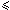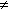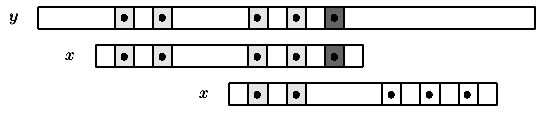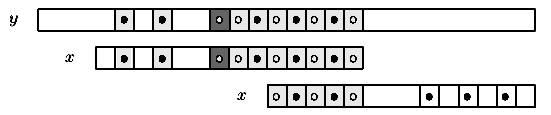Name
• String
• Substring
• Search
Edit
For 0im-1: kmin[i]= d>0 iff x[0 .. i-1-d]=x[d .. i-1] and x[i-d]x[i], 0 otherwise.

When kmin0 a periodicity ends at position i in x.

For 0 < i < m if kmin[i-1]  0 then i is a nohole otherwise i is a hole.

Let nd+1 be the number of noholes in x.
The table h contains first the nd+1 noholes in increasing order and then the m-nd-1 holes in decreasing order:

• for 0ind, h[i] is a nohole and h[i] < h[i+1] for 0i<nd;
• for nd < i < m, h[i] is a hole and h[i] > h[i+1] for nd < i < m-1.

If i is a hole then rmin[i] is the smallest period of x greater than i.

The value of first[u] is the smallest integer v such that uh[v].

Then assume that x is aligned with y[j .. j+m-1]. If x[h[k]]=y[j+h[k]] for 0k < r < nd and x[h[r]]y[j+h[r]]. Let j’ = j+kmin[h[r]].
Then there is no occurrence of x beginning in y[j .. j’] and x can be shifted by kmin[h[r]] positions to the right.
Moreover x[h[k]]=y[j’+h[k]] for 0  k < first[h[r]-kmin[h[r]]] meaning that the comparisons can be resume with x[h[first[h[r]-kmin[h[r]]]]] and y[j’+h[first[h[r]-kmin[h[r]]]]].Figure 9.1: Mismatch with a nohole. Noholes are black circles and are compared from left to right.
In this situation, after the shift, it is not necessary to compare the first two noholes again.

If x[h[k]]=y[j+h[k]] for 0k < r and x[h[r]]y[j+h[r]] with nd  r < m. Let j’=j+rmin[h[r]]. Then there is no occurrence of x beginning in y[j .. j’] and x can be shifted by kmin[h[r]] positions to the right.
Moreover x[0 .. m-1-rmin[h[r]]]=y[j’ .. j+m-1] meaning that the comparisons can be resume with x[h[first[m-1-rmin[h[r]]]]] and y[j’+h[first[m-1-rmin[h[r]]]]].Figure 9.2: Mismatch with a hole. Noholes are black circles and are compared from left to right
while holes are white circles and are compared from right to left.
In this situation, after the shift, it is not necessary to compare the matched prefix of the pattern again.

To compute the values of kmin, a table hmax is used and defined as follows: hmax[k] is such that x[k .. hmax[k]-1]=x[0 .. hmax[k]-k-1] and x[hmax[k]]x[hmax[k]-k].

The value of nhd0[i] is the number of noholes strictly smaller than i.
We can now define two functions shift and next as follows:

• shift[i]=kmin[h[i]] and next[i]=nhd0[h[i]-kmin[h[i]]] for i < nd;
• shift[i]=rmin[h[i]] and next[i]=nhd0[m-rmin[h[i]]] for ndi < m;
• shift[m]=rmin and next[m]=nhd0[m-rmin[h[m-1]]].

Thus, during an attempt where the window is positioned on the text factor y[j .. j+m-1], when a mismatch occurs between x[h[r]] and y[j+h[r]] the window must be shifted by shift[r] and the comparisons can be resume with pattern position h[next[r]].

The preprocessing phase can be done in O(m) space and time. The searching phase can then be done in O(n) time complexity and furthermore at most n text character comparisons are performed during the searching phase.
C